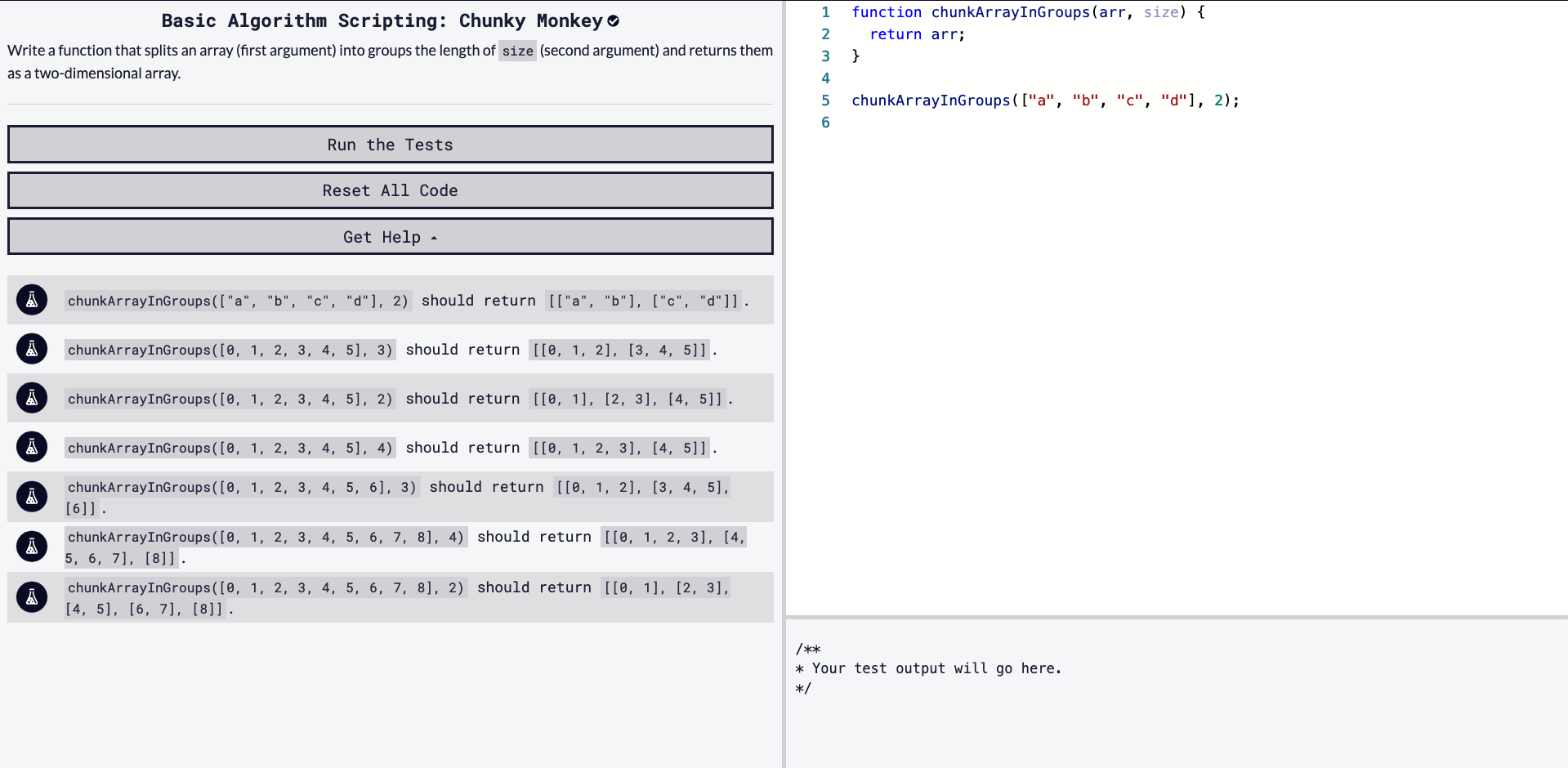## DEV Community

Viren B

Posted on • Originally published at virenb.cc

# Solving "Chunky Monkey" / freeCodeCamp Algorithm ChallengesLet's solve freeCodeCamp's basic algorithm scripting challenge, 'Chunky Monkey'.

### Starter Code

``````function chunkArrayInGroups(arr, size) {
return arr;
}

chunkArrayInGroups(["a", "b", "c", "d"], 2);
``````

### Instructions

Write a function that splits an array (first argument) into groups the length of `size` (second argument) and returns them as a two-dimensional array.

### Tests

``````chunkArrayInGroups(["a", "b", "c", "d"], 2) should return [["a", "b"], ["c", "d"]].
chunkArrayInGroups([0, 1, 2, 3, 4, 5], 3) should return [[0, 1, 2], [3, 4, 5]].
chunkArrayInGroups([0, 1, 2, 3, 4, 5], 2) should return [[0, 1], [2, 3], [4, 5]].
chunkArrayInGroups([0, 1, 2, 3, 4, 5], 4) should return [[0, 1, 2, 3], [4, 5]].
chunkArrayInGroups([0, 1, 2, 3, 4, 5, 6], 3) should return [[0, 1, 2], [3, 4, 5], ].
chunkArrayInGroups([0, 1, 2, 3, 4, 5, 6, 7, 8], 4) should return [[0, 1, 2, 3], [4, 5, 6, 7], ].
chunkArrayInGroups([0, 1, 2, 3, 4, 5, 6, 7, 8], 2) should return [[0, 1], [2, 3], [4, 5], [6, 7], ].
``````

# Our Approach

Read everything first. Read the instructions clearly, read the starter code we're given, and read the tests and understand what has to be returned.

• The function takes two arguments, `arr` being an array and `size` being a number.
• We need to evaluate `size` and create sub-arrays with a length of `size`.
• We need to return a two-dimensional array.

Now that we understand what we are given and what we want to output, let's see how we can solve this.

Our plan of action will definitely involving a loop of `arr`. We need to figure out how and when to split into a sub-array.

From previous challenges, we know that `splice()` method can alter our `arr` and return a new array.

MDN: Array.splice()

Just a quick reminder of how `splice()` works:

"The splice() method changes the contents of an array by removing or replacing existing elements and/or adding new elements in place."

``````// Let's delete 3, 4
[1, 2, 3, 4].splice(2, 2)
Going to 2nd index and removing 2 items
[3, 4]
``````

Since `splice()` returns an array, we can save it with a variable, then insert it back into `arr`.

We have a better idea on slicing now, we can create our for loop but how should we call `slice()`?

``````for (let i = 0; i < arr.length; i++) {
let toSplice = arr.splice(i, size)
...
}
``````

We will call splice with `splice(i, size)` as size is the value of how big our new arrays should be.

Taking a test case for example:

``````chunkArrayInGroups([0, 1, 2, 3, 4, 5], 3) should return [[0, 1, 2], [3, 4, 5]

for (let i = 0; i < arr.length; i++) {
let toSplice = arr.splice(i, size)
...
}

First loop:
i = 0
size = 3
toSplice = [0, 1, 2]
arr = [3, 4, 5]

Second loop:
i = 1
size = 3
toSplice = [4, 5]
arr = 
``````

So, we are on the right track but on the second loop, it starts to take the wrong direction.

Another Array method will be of much use now, `unshift()`.

"The unshift() method adds one or more elements to the beginning of an array and returns the new length of the array."

MDN: Array.unshift()

We want to splice, then unshift.

`arr.unshift(toSplice);`

It will add the new array to the front of `arr`.

Once we add `unshift()`, our first loop will look like the following:

``````chunkArrayInGroups([0, 1, 2, 3, 4, 5], 3) should return [[0, 1, 2], [3, 4, 5]

for (let i = 0; i < arr.length; i++) {
let toSplice = arr.splice(i, size);
arr.unshift(toSplice);
}

First loop:
i = 0
size = 3
toSplice = [0, 1, 2]
arr = [[0, 1, 2], 3, 4, 5]

``````

I would call this sucessful. We are slowly creating out desired 2D array.

Our second loop would look like:

``````Second loop:
i = 1
size = 3
toSplice = [3, 4, 5]
arr = [ [ 3, 4, 5 ], [ 0, 1, 2 ] ]
``````

The only issue is the sub-arrays are in reverse order.

We have another method we can use, `reverse()`.

``````[[ 3, 4, 5 ], [ 0, 1, 2 ] ].reverse()
Result: [[0, 1, 2], [3, 4, 5]]
``````

Always make sure to return!

# Our Solution [SPOILER: CONTAINS ANSWER]

``````function chunkArrayInGroups(arr, size) {
for (let i = 0; i < arr.length; i++) {
let toSplice = arr.splice(i, size);
arr.unshift(toSplice);
}
return arr.reverse();
}
``````